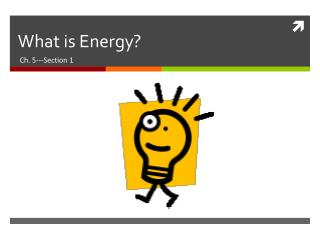DownloadDownload PresentationWhat is Energy?

# What is Energy?

Télécharger la présentation## What is Energy?

- - - - - - - - - - - - - - - - - - - - - - - - - - - E N D - - - - - - - - - - - - - - - - - - - - - - - - - - -
##### Presentation Transcript

1. What is Energy? Ch. 5---Section 1

2. Energy • The ability to cause change • Can take many different forms • Kinetic - moving • Potential - positional • Thermal – Increases with heat • Chemical – stored in bonds • Radiant – light • Sound – vibration of molecules • Electrical – from electricity • Nuclear – stored in atoms • SI unit is the joule (J) • Energy and work are related • Energy is the ability to do work and work is the transfer of energy, therefore they are measured the same way!

3. Kinetic Energy (KE) • The energy an object has due to its motion • If an object isn’t moving it doesn’t have any KE • Depends on both the mass and velocity of an object: • If two objects have the same mass, the one travelling with greater velocity has more KE • If two objects are travelling the same velocity, the object with greater mass has more KE

4. Gravitational Potential Energy (GPE) • The energy an object has due to its position • If an object isn’t moving, it can still have GPE • Depends on both the mass of the object and it’s height above the ground: • If two objects have the same mass, the one that is higher up has more GPE • If two objects are positioned at the same height, the object with greater mass has more GPE • KE and GPE are collectively referred to as Mechanical Energy

5. Potential Vs. Kinetic Energy • Law of conservation of energy - energy cannot be created or destroyed. 1. Potential energy = 50 J Kinetic energy = 0 J Potential energy = 25 J Kinetic energy = 25 J 2. Potential energy = 0 J Kinetic energy = 50 J 3.

6. Thermal Energy • The energy that all objects have that increases when the object’s temperature increases • Due to the motion of molecules that make up the object • Ex. A cup of boiling water has more thermal energy than a cup of cold water, which has more thermal energy than a cup of ice. • Usually is the last step in all energy transformations because it is so hard to store.

7. Chemical Energy • The energy that is stored in chemical bonds • Is released when bonds between compounds are broken • Examples of substances that contain chemical energy: • Wood • Gasoline • Food

8. Radiant Energy (Light Energy) • Energy that is carried by light • Can be converted into thermal energy to warm objects • Light travels at 300,000 km/s, which is fast enough to circle the Earth eight times in one second.

9. Sound Energy • Energy that is transmitted through a medium in the form of vibrations. • Is a mechanical wave – requires material to travel through…cannot travel through a vacuum (No sound in space) • The denser the material, the faster sound will travel (travels fastest in solids)

10. Electrical Energy • Energy carried by an electrical current • Caused by fast moving particles • These particles have charges that attract or repel each other.

11. Nuclear Energy • Energy contained in the nucleus of an atom • Can be released two ways: • fission • fusion